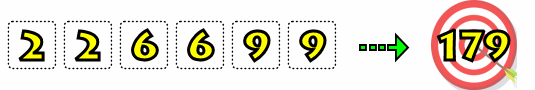# Target Number #31- Make 179 -Using the numbers 2, 2, 6, 6, 9, and 9 (in any order), can you write a mathematical expression (e.g. (6*2)*(9+9)-(6/2)) with a result equal to 179?

You may only use the four BASIC math operations (addition, subtraction, multiplication, division) as well as parenthesis. Some additional rules:

1. You are not required to use all six numbers.
2. You may NOT join numbers (e.g. 9 and 6 to make 96).
3. You may only use a number as many times as it appears above.Need a Hint?
Do you have a suggestion for this puzzle (e.g. something that should be mentioned/clarified in the question or solution, bug, typo, etc.)?
• Home
• Puzzle Categories
• Monthly Contest
• Everything Else